Theory and Modern Applications

# Soliton solutions of q-Toda lattice by Hirota direct method

## Abstract

This paper presents the q-analogue of Toda lattice system of difference equations by discussing the q-discretization in three aspects: differential-q-difference, q-difference-q-difference and q-differential-q-difference Toda equation. The paper develops three-q-soliton solutions, which are expressed in the form of a polynomial in power functions, for the differential-q-difference and q-difference-q-difference Toda equations by Hirota direct method. Furthermore, it introduces q-Hirota D-operator and presents the q-differential-q-difference version of Toda equation. Finally, the paper presents its solitary wave like a solution in terms of q-exponential function and explains the nonexistence of further solutions in terms of q-exponentials by the virtue of Hirota perturbation.

## 1 Introduction

In the literature, to find exact solutions of nonlinear partial differential equations, a vast variety of methods have been proposed such as the inverse scattering transform , Bäcklund transformation , Hirota direct method and several dressing methods. Among these methods, Hirota direct method, introduced in the pioneering article , is one of the most effective and fastest methods for constructing not only multi-soliton solutions but also some special solutions of integrable nonlinear evolution equations. The method has been shown to be applicable to a wide class of equations including nonlinear differential, nonlinear differential-difference and nonlinear difference equations . The first step of the method is to utilize a dependent variable transformation to convert the nonlinear partial differential or difference equation into a quadratic form, the so-called bilinear form, in the new dependent variables. The fundamental idea behind the method is to write the bilinear form as a polynomial of Hirota-D operator (derivative). This compact form is called a Hirota bilinear form. It should be noted that it is not possible to give an algorithm to write every nonlinear partial differential or difference equation in a Hirota bilinear form. Besides some equations may not have Hirota bilinear forms but trilinear or multilinear forms . It is conjectured that all completely integrable nonlinear partial differential or difference equations can be written in a Hirota bilinear form. On the other hand, for an equation admitting a Hirota bilinear form does not guarantee the existence of N-soliton solutions of any order. The equations admitting a Hirota bilinear form and having 3-soliton solutions are said to be Hirota integrable. Such equations together with Painlevé approach are widely believed to be integrable. Therefore, Hirota direct method builds a bridge between having multi-soliton solutions and the theory of integrability, in the sense that the so-called Hirota condition (three-soliton solution condition) is a milestone for investigating the integrability of an equation . The rest of the method is to use a perturbation expansion (also known as Hirota perturbation) in a Hirota bilinear form, collect the coefficients of perturbation parameter and analyze the conditions for multi-soliton solutions.

The new dependent variables (the so-called τ-functions) are essential in the literature. They are expressed as polynomials in exponential functions in Hirota perturbation while in Sato theory they are written in terms of Wronski or Casorati determinants providing solutions to equations in bilinear forms [11, 12].

In , a general framework of integrable discrete systems on $\mathbb{R}$ is introduced by the virtue of discrete one-parameter group of diffeomorphisms. These diffeomorphisms are expressed by infinitesimal generators $\chi \left(x\right){\partial }_{x}$, where in particular, $\chi =1$ corresponds to Toda lattice while $\chi =x$ subtends to q-deformed Toda lattice. It is concluded that all discrete systems determined by different vector fields $\chi \left(x\right){\partial }_{x}$ are not globally equivalent, moreover q-difference systems on ${\mathbb{R}}^{-}$ are not isomorphic to lattice systems on $\mathbb{R}$.

Inspired by this fact, the purpose of this paper is to present the q-analogue of Toda lattice system of difference equations and discuss the applicability of Hirota direct method for constructing multi-soliton solutions. There are several ways to q-discretize a given continuous equation. By q-discretization we mean q-difference equations determined by q-difference operator and additionally q-differential equations constructed by q-derivative operator. Therefore, we present q-discretization in three aspects: differential-q-difference, q-difference-q-difference and q-differential-q-difference Toda equation. We show that Hirota direct method allows to produce three-soliton solutions for the differential-q-difference and q-difference-q-difference Toda equations. We emphasize that the solutions not only possess soliton behaviors but have additional power counterparts for q-discrete variables. We call such soliton solutions as q-soliton solutions. Therefore, unlike the Toda equation  or discrete-time Toda equation , the differential-q-difference and q-difference-q-difference Toda equations have soliton solutions in the form of a polynomial in power functions. On the other hand, we conclude that Hirota direct method fails to derive multi-soliton solutions of the q-differential-q difference Toda equation. Furthermore, it is not possible to obtain multi-soliton solutions for any q-differential-q-difference or q-differential-difference type of equations by means of Hirota perturbation.

In Section 3, we introduce the q-analogue of the exponential identity in order to overcome the obstacles in front of deriving a Hirota bilinear form for q-discrete equations. This exponential identity arises in terms of forward and backward shift not on the level of additive sense but multiplicative sense. In Section 4, we propose the differential-q-difference Toda equation. Since Hirota condition (three-soliton solution condition) is an indicator for integrability, we present three-q-soliton solutions of the differential-q-difference Toda equation. In Section 5, reversing the procedure, we introduce a proper Hirota bilinear form from which we construct q-difference-q-difference Toda equation and its three-q-soliton solutions. In the last section, we introduce and develop the notion of q-Hirota D-operator. We present the q-differential-q-difference version of Toda equation and construct its Hirota bilinear form in terms of q-Hirota D-operator. We present its solitary wave like a solution in terms of q-exponential function and explain the nonexistence of further q-exponential type solutions by Hirota perturbation.

## 2 Basic notions

In order to obtain the solutions of nonlinear partial differential or difference equations

$F\left[u\right]=F\left(u,{u}_{x},{u}_{t},\dots \right)=0,$
(1)

Hirota direct method allows to write such equations in a form where the new dependent variables appear bilinearly by using suitable bilinearizing transformations

$u=T\left[f\left(x,t,\dots \right)\right].$
(2)

This form is called bilinear form of $F\left[u\right]$. We should remark that some integrable equations can only be transformed to a single bilinear form while some of them can be written as a combination of bilinear forms. On the other hand, for some equations it is not possible to find a proper transformation. The next step towards Hirota direct method, is introducing the so-called Hirota D-operator which is a binary differential operator exhibiting a new calculus.

Definition 2.1 Let S be a space of differentiable functions $f:{\mathbb{C}}^{n}\to \mathbb{C}$. The Hirota D-operator $D:S×S\to S$ is defined as

$\left[{D}_{x}^{{m}_{1}}{D}_{t}^{{m}_{2}}\cdots \right]\left\{f\cdot g\right\}=\left[{\left({\partial }_{x}-{\partial }_{{x}^{\prime }}\right)}^{{m}_{1}}{\left({\partial }_{t}-{\partial }_{{t}^{\prime }}\right)}^{{m}_{2}}\cdots \right]f\left(x,t,\dots \right)g\left({x}^{\prime },{t}^{\prime },\dots \right){|}_{{x}^{\prime }=x,{t}^{\prime }=t,\dots },$
(3)

where $x,t,\dots$ are independent variables and ${m}_{i}\in {\mathbb{Z}}^{+}$, $\mathrm{\forall }i⩾1$.

The core of the method is to construct the so-called Hirota bilinear form, which is a compact form of the bilinear form of $F\left[u\right]$, expressed in terms of a polynomial of Hirota-D operator. The last step towards the method is the application of the Hirota perturbation. We plug the finite perturbation expansion of the dependent variables $f\left(x,t,\dots \right)$ into a Hirota bilinear form. We examine the coefficients of the perturbation parameter and analyze the conditions for multi-soliton solutions. We finish this section by summing up the properties of the Hirota D-operator (3) .

Proposition 2.2 Let $P\left(D\right)$be an arbitrary polynomial in D acting on two differentiable functions$f\left(x,t,\dots \right)$and$g\left(x,t,\dots \right)$, then the following equations hold:

$\left(i\right)\phantom{\rule{1em}{0ex}}P\left(D\right)\left\{f\cdot g\right\}=P\left(-D\right)\left\{gf\right\},$
(4)
$\left(ii\right)\phantom{\rule{1em}{0ex}}P\left(D\right)\left\{f\cdot 1\right\}=P\left(\partial \right)f;P\left(D\right)\left\{1\cdot f\right\}=P\left(-\partial \right)f,$
(5)

where is the usual differential operator.

## 3 q-exponential identity

It is possible to introduce Hirota D-operator by the virtue of the exponential identity

$exp\left(h{D}_{x}\right)f\left(x\right)g\left(x\right)=f\left(x+h\right)g\left(x-h\right),$
(6)

where h is a parameter and $f,g$ are continuously differentiable functions to all orders in x. In this section, our aim is to introduce the q-analogue of the exponential identity (6). For this purpose, we first explain its construction by using the notions presented in . Let $\sigma :\mathbb{R}\to \mathbb{R}$ and $\rho :\mathbb{R}\to \mathbb{R}$ be the forward and backward jump operators, respectively. Here, in $m\in {\mathbb{Z}}^{+}$ forward steps, $x\in \mathbb{R}$ is mapped to ${\sigma }_{m}\left(x\right)$, where ${\sigma }_{m}$ is the m-times composition of σ, and in m backward steps x is mapped to ${\rho }_{m}\left(x\right)$. If there exist inverse maps ${\sigma }^{-1}$ and ${\rho }^{-1}$, such that $\sigma \left(x\right)={\rho }^{-1}\left(x\right)$ and $\rho \left(x\right)={\sigma }^{-1}\left(x\right)$ for all $x\in \mathbb{R}$, then bijective σ defines a discrete one-parameter group of bijections on $\mathbb{R}$: $\mathbb{Z}\ni m\to \left\{{\sigma }_{m}:\mathbb{R}\to \mathbb{R}\right\}$, where ${\sigma }_{0}\equiv {\mathrm{id}}_{\mathbb{R}}$. Since infinite-dimensional systems of smooth dynamical fields are under consideration, it is better to introduce one-parameter group of diffeomorphisms instead of bijections. Consider the continuous one-parameter group of diffeomorphisms $\mathbb{R}\ni t↦{\sigma }_{t}$. By Taylor expansion of ${\sigma }_{t}$ around $t=0$,

${\sigma }_{t}\left(x\right)=x+t\cdot \frac{d{\sigma }_{t}\left(x\right)}{dt}{|}_{t=0}+O\left({t}^{2}\right),$

it is clear that one-parameter group of diffeomorphism is generated by a vector field (the so-called infinitesimal generator), denoted by $\mathcal{X}\left(x\right){\partial }_{x}$, i.e., ${\sigma }_{t}\left(x\right)=x+t\cdot \mathcal{X}\left(x\right)+O\left({t}^{2}\right)$, where $\mathcal{X}\left(x\right)$ is smooth on $\mathbb{R}$ except at most finite number of points. The computation of one-parameter group generated by a vector field is often referred as exponentiation of the vector field, therefore we have

${\sigma }_{h}\left(x\right)={e}^{h\chi \left(x\right){\partial }_{x}}x\phantom{\rule{1em}{0ex}}⇔\phantom{\rule{1em}{0ex}}{e}^{h\chi \left(x\right){\partial }_{x}}u\left(x\right)=u\left({e}^{h\chi \left(x\right){\partial }_{x}}x\right)=u\left({\sigma }_{h}\left(x\right)\right),$
(7)

where $u\left(x\right)$ is a smooth function and $h>0$ is some deformation parameter. If we consider the vector fields of the form $\chi \left(x\right){\partial }_{x}={x}^{1-n}{\partial }_{x}$ on $\mathbb{R}$, for $n=0$, we have

${\sigma }_{h}\left(x\right)={e}^{hx{\partial }_{x}}x={e}^{h}x=qx,\phantom{\rule{2em}{0ex}}q\equiv {e}^{h}\phantom{\rule{1em}{0ex}}⇔\phantom{\rule{1em}{0ex}}{e}^{hx{\partial }_{x}}u\left(x\right)=u\left(qx\right),$
(8)

which corresponds to the q-difference type of discussions. For $n=1$, we deal with the case of lattice type

${\sigma }_{h}\left(x\right)={e}^{h{\partial }_{x}}x=x+h\phantom{\rule{1em}{0ex}}⇔\phantom{\rule{1em}{0ex}}{e}^{h{\partial }_{x}}u\left(x\right)=u\left(x+h\right).$

All discrete systems defined by different vector fields $\chi \left(x\right){\partial }_{x}$ are not globally equivalent. However, it is possible to find a local transformation relating these vector fields. If we consider $\chi \left(x\right)={x}^{1-n}$ where n ($n\ne 0$) is odd, and ${\chi }^{\prime }\left({x}^{\prime }\right)=1$, there exists a bijection ${x}^{\prime }=\frac{1}{n}{x}^{n}$ on $\mathbb{R}-\left\{0\right\}$. Therefore all discrete systems generated by $\chi \left(x\right){\partial }_{x}={x}^{1-n}{\partial }_{x}$ (n is odd), can be reduced to the original lattice Toda-type systems with $x\ne 0$. However, if we consider $\chi \left(x\right)=x$ (q-discrete case) and ${\chi }^{\prime }\left({x}^{\prime }\right)=1$, we have $x={e}^{{x}^{\prime }}$ which is not a bijection provided that $x\notin {\mathbb{R}}^{+}$(see  for more precise details).

As a result, q-difference systems on ${\mathbb{R}}^{-}$, determined by q-shift operators ${E}_{q}:={e}^{hx{\partial }_{x}}$ are not isomorphic to lattice systems on $\mathbb{R}$. Motivated by this in-equivalence, in order to examine how soliton solutions of q-difference systems differ from soliton solutions of discrete systems, we introduce the following q-exponential identity. Such q-exponential identity shed light to construct Hirota bilinear forms of q-discrete equations under consideration.

Theorem 3.1 For arbitrary continuously differentiable functions$f\left(x\right)$, $g\left(x\right)$, the q-exponential identity

${e}^{hx{D}_{x}}f\left(x\right)g\left(x\right)=f\left(qx\right)g\left(\frac{x}{q}\right)={E}_{q}f\left(x\right){E}_{q}^{-1}g\left(x\right),\phantom{\rule{1em}{0ex}}x\in \mathbb{R},$
(9)

holds where we have the usual relation between two quantum parameters h and q as$q={e}^{h}$. Here${E}_{q}$and${E}_{q}^{-1}$are q-forward and backward jump operators, respectively acting as

${E}_{q}f\left(x\right)=f\left(qx\right),\phantom{\rule{2em}{0ex}}{E}_{q}^{-1}f\left(x\right)=f\left(\frac{x}{q}\right).$
(10)

Proof First, we note that for the left hand side of the identity (9), we are not able to expand the exponential operator since ${D}_{x}$ is not an associative bilinear operation. Besides, for the right hand side, the Taylor series expansions are not valid either because of the fact that we seek for forward and backward shifting not on the level of additive sense but multiplicative sense. Therefore, the only way to prove the identity is to make use of the change of variable $x{D}_{x}={D}_{{x}^{\prime }}$ which implies $x={e}^{{x}^{\prime }}$ by integration with respect to x. Here integration constant is ignored since it would be embedded while the operator ${D}_{x}$ acts in a Hirota bilinear form. Then

${e}^{hx{D}_{x}}f\left(x\right)g\left(x\right)={e}^{h{D}_{{x}^{\prime }}}f\left({e}^{{x}^{\prime }}\right)g\left({e}^{{x}^{\prime }}\right).$
(11)

Using (6) for the right hand side of (11), we end up with

${e}^{hx{D}_{x}}f\left(x\right)g\left(x\right)=f\left({e}^{{x}^{\prime }+h}\right)g\left({e}^{{x}^{\prime }-h}\right)=f\left({e}^{{x}^{\prime }}{e}^{h}\right)g\left({e}^{{x}^{\prime }}{e}^{-h}\right)=f\left(qx\right)g\left(\frac{x}{q}\right)={E}_{q}f\left(x\right){E}_{q}^{-1}g\left(x\right).$

□

We finish this section by defining the following difference operator.

Definition 3.2 We define the central q-difference operator ${\mathrm{\Delta }}_{x}^{2}$ operating on an arbitrary function $f\left(x\right)$, $x\in \mathbb{R}$, as

${\mathrm{\Delta }}_{x}^{2}f\left(x\right)=f\left(qx\right)+f\left(\frac{x}{q}\right)-2f\left(x\right),\phantom{\rule{1em}{0ex}}q\ne 1.$
(12)

Note that throughout the forthcoming framework, we may suppose $q>1$. One can rewrite (12), by using the q-forward and backward jump operators (10) as

${\mathrm{\Delta }}_{x}^{2}f\left(x\right)=\left({E}_{q}+{E}_{q}^{-1}-2\right)f\left(x\right).$

## 4 The differential-q-difference Toda equation

Toda equation  which describes the motions of anharmonic one-dimensional lattice is given by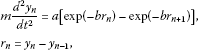(13)

where a, b and m are real constants. Introducing the force of the n th spring in the lattice

${V}_{n}=a\left[exp\left(-b{r}_{n}\right)-1\right],$
(14)

as a rapidly decaying function, the equation (13) turns out to be

$\frac{{d}^{2}}{d{t}^{2}}log\left(1+{V}_{n}\right)={V}_{n+1}+{V}_{n-1}-2{V}_{n}.$
(15)

In this section, our aim is to establish the three-soliton solutions of the differential-q-difference Toda equation proposed as follows:

$\frac{{d}^{2}}{d{t}^{2}}log\left(1+V\left(x,t\right)\right)={\mathrm{\Delta }}_{x}^{2}V\left(x,t\right)=V\left(qx,t\right)+V\left(\frac{x}{q},t\right)-2V\left(x,t\right).$
(16)

Let us introduce the dependent variable transformation as

$V\left(x,t\right)=\frac{{d}^{2}}{d{t}^{2}}logf\left(x,t\right).$
(17)

If we integrate (16) twice with respect to time variable t, make use of the transformation (17) in (16) and impose the condition that V is a rapidly decaying function, we evolve the bilinear form for $f\left(x,t\right)$

$V\left(x,t\right)=\frac{{f}_{tt}f-{f}_{t}^{2}}{{f}^{2}}=\frac{f\left(qx,t\right)f\left(\frac{x}{q},t\right)}{{f}^{2}}-1.$
(18)

One can derive a Hirota bilinear form, namely the bilinear form in terms of Hirota D-operator, as

$\left[{{D}_{t}}^{2}-\left(exp\left(hx{D}_{x}\right)+exp\left(-hx{D}_{x}\right)-2\right)\right]\left\{f\left(x,t\right)\cdot f\left(x,t\right)\right\}=0,$
(19)

what follows from (18) by multiplying with $2{f}^{2}\left(x,t\right)$, where we utilize the q-exponential identity (9). In order to obtain three-soliton solutions, we make use of finite perturbation expansion around a formal perturbation parameter ε, as

$f\left(x,t\right)=1+\epsilon {f}^{\left(1\right)}\left(x,t\right)+{\epsilon }^{2}{f}^{\left(2\right)}\left(x,t\right)+\cdots .$
(20)

Substituting in (20)

$P\left(D\right)\left\{f\left(x,t\right)\cdot f\left(x,t\right)\right\}=0,$
(21)

we have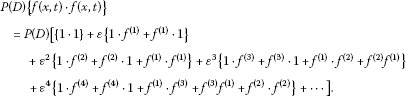(22)

Here $P\left(D\right)$ is given by (19). We collect the coefficients of ${\epsilon }^{i}$, $\mathrm{\forall }i⩾0$. The coefficient of the first term ${\epsilon }^{0}$ vanishes trivially, while from the coefficient of ${\epsilon }^{1}$, we have

$P\left(D\right)\left\{1\cdot {f}^{\left(1\right)}+{f}^{\left(1\right)}\cdot 1\right\}=2P\left(\partial \right){f}^{\left(1\right)}=2\left[{\partial }_{t}^{2}-\left(exp\left(hx{\partial }_{x}\right)+exp\left(-hx{\partial }_{x}\right)-2\right)\right]{f}^{\left(1\right)}=0,$
(23)

which is a direct result of the property (5) of D operator, since $P\left(D\right)$ is of even order. We need to seek for a solution for the equation (23).

Remark 4.1 The general tendency for soliton solutions is being of exponential type; however, such an exponential function ${f}^{\left(1\right)}$ does not satisfy the equation (23). Because of the nature of the q-numbers, the solution of the equation (23) should have a power function for the q-discrete space variable counterpart. Therefore, one may choose the starting solution of (23) as

${f}^{\left(1\right)}\left(x,t\right)={x}^{\alpha }exp\left(\beta t+\eta \right),$
(24)

where $\alpha ,\eta$ are arbitrary constants. Indeed, such an inevitable choice is a direct consequence of change of variable ${\partial }_{y}=x{\partial }_{x}$.

Hereafter, throughout the rest of the discussions, we give the following definition.

Definition 4.2 A solution possessing usual soliton behaviors and having power counterparts for q-discrete variables is called a q-soliton solution.

If we substitute (24) in (23), we derive the relation among the parameters

${\beta }^{2}={q}^{\alpha }+{q}^{-\alpha }-2,$
(25)

which is said to be dispersion relation. The coefficient of ${\epsilon }^{2}$, resulting from (22), yields as

$P\left(D\right)\left\{1\cdot {f}^{\left(2\right)}+{f}^{\left(2\right)}\cdot 1+{f}^{\left(1\right)}\cdot {f}^{\left(1\right)}\right\}=2P\left(\partial \right){f}^{\left(2\right)}+P\left(D\right)\left\{{f}^{\left(1\right)}\cdot {f}^{\left(1\right)}\right\},$

which implies explicitly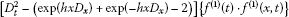(26)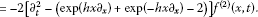(27)

We note that since ${f}^{\left(1\right)}$ given in (24) satisfies the form (26), we are allowed to assume all higher order terms to be taken zero, i.e., ${f}^{\left(j\right)}=0$, $j⩾2$. Thus, hereafter as a generalization of this fact, one can assume in deriving i-q-soliton solution, ${f}^{\left(j\right)}=0$ for all $j⩾i+1$. Without loss of generality, we may set $\epsilon =1$; and therefore, we construct one-q-soliton solution using (18) by the virtue of (24) and (25) as

$V\left(x,t\right)=\frac{{\beta }^{2}{x}^{\alpha }exp\left(\beta t+\eta \right)}{{\left(1+{x}^{\alpha }exp\left(\beta t+\eta \right)\right)}^{2}}.$
(28)

For the two-q-soliton solutions, we choose the starting solution of (23) as

${f}^{\left(1\right)}={x}^{{\alpha }_{1}}exp\left({\beta }_{1}t+{\eta }_{1}\right)+{x}^{{\alpha }_{2}}exp\left({\beta }_{2}t+{\eta }_{2}\right),$
(29)

where ${\alpha }_{i}$, ${\eta }_{i}$ are arbitrary constants $\mathrm{\forall }i=1,2$. Similarly, collecting the coefficients of ${\epsilon }^{i}$, $\mathrm{\forall }i⩾0$, the coefficient of ${\epsilon }^{0}$ vanishes trivially and from the coefficient of ${\epsilon }^{1}$, we obtain the related dispersion relation

${\beta }_{i}^{2}={q}^{{\alpha }_{i}}+{q}^{-{\alpha }_{i}}-2,\phantom{\rule{1em}{0ex}}\mathrm{\forall }i=1,2.$
(30)

Here we remark that the use of vector notation

${p}_{1}±{p}_{2}=\left({\beta }_{1}±{\beta }_{2},{\alpha }_{1}±{\alpha }_{2},{\eta }_{1}±{\eta }_{2}\right),$
(31)

leads to rewrite dispersion relation (30) as $P\left(pi\right)=0$, for all $i=1,2$. Subsequently, the coefficient of ${\epsilon }^{2}$ implies

$-P\left(\partial \right){f}^{\left(2\right)}=\left[{\left({\beta }_{1}-{\beta }_{2}\right)}^{2}-\left({q}^{{\alpha }_{1}-{\alpha }_{2}}+{q}^{{\alpha }_{2}-{\alpha }_{1}}-2\right)\right]{x}^{{\alpha }_{1}+{\alpha }_{2}}exp\left(\left({\beta }_{1}+{\beta }_{2}\right)t+\left({\eta }_{1}+{\eta }_{2}\right)\right).$
(32)

Thus, ${f}^{\left(2\right)}$ is of the form

${f}^{\left(2\right)}=A\left(1,2\right){x}^{{\alpha }_{1}+{\alpha }_{2}}exp\left(\left({\beta }_{1}+{\beta }_{2}\right)t+\left({\eta }_{1}+{\eta }_{2}\right)\right).$
(33)

Plugging such ${f}^{\left(2\right)}$ into (32), one can obtain the interaction term which determines the change of position resulting from the interaction of two-q-solitons as

$A\left(1,2\right)=-\frac{{\left({\beta }_{1}-{\beta }_{2}\right)}^{2}-\left({q}^{{\alpha }_{1}-{\alpha }_{2}}+{q}^{{\alpha }_{2}-{\alpha }_{1}}-2\right)}{{\left({\beta }_{1}+{\beta }_{2}\right)}^{2}-\left({q}^{{\alpha }_{1}+{\alpha }_{2}}+{q}^{-{\alpha }_{1}-{\alpha }_{2}}-2\right)}=-\frac{P\left({p}_{1}-{p}_{2}\right)}{P\left({p}_{1}+{p}_{2}\right)}.$
(34)

Since ${f}^{\left(3\right)}=0$, by the use of the dispersion relation (30) the coefficient of ${\epsilon }^{3}$ vanishes trivially, so do the rest of ${\epsilon }^{j}$, $\mathrm{\forall }j>3$. Thus, the solution describing a head collision of two-q-solitons (see Figure 1) is expressed as

$\begin{array}{rcl}f\left(x,t\right)& =& 1+{x}^{{\alpha }_{1}}exp\left({\beta }_{1}t+{\eta }_{1}\right)+{x}^{{\alpha }_{2}}exp\left({\beta }_{2}t+{\eta }_{2}\right)\\ +A\left(1,2\right){x}^{{\alpha }_{1}+{\alpha }_{2}}exp\left(\left({\beta }_{1}+{\beta }_{2}\right)t+\left({\eta }_{1}+{\eta }_{2}\right)\right).\end{array}$
(35)

One can derive the three-q-soliton solution, by choosing the starting solution of (23) as

${f}^{\left(1\right)}=\sum _{i=1}^{3}{x}^{{\alpha }_{i}}exp\left({\beta }_{i}t+{\eta }_{i}\right),$
(36)

where ${\alpha }_{i}$, ${\eta }_{i}$ are arbitrary constants $\mathrm{\forall }i=1,2,3$. Similar to the previous arguments, the coefficient of ${\epsilon }^{0}$ vanishes trivially. From the coefficient of ${\epsilon }^{1}$, we have the corresponding dispersion relation

${\beta }_{i}^{2}={q}^{{\alpha }_{i}}+{q}^{-{\alpha }_{i}}-2,\phantom{\rule{1em}{0ex}}\mathrm{\forall }i=1,2,3,$
(37)

while from the coefficient of ${\epsilon }^{2}$, we obtain

$-P\left(\partial \right){f}^{\left(2\right)}=\sum _{i
(38)

where the summation is over all possible pairs of three elements such that $i. The equation (38) implies the explicit form of ${f}^{\left(2\right)}$

${f}^{\left(2\right)}=\sum _{i
(39)

where the interaction terms among three-q-soliton solutions are

$A\left(i,j\right)=-\frac{P\left({p}_{i}-{p}_{j}\right)}{P\left({p}_{i}+{p}_{j}\right)}=-\frac{{\left({\beta }_{i}-{\beta }_{j}\right)}^{2}-\left({q}^{{\alpha }_{i}-{\alpha }_{j}}+{q}^{{\alpha }_{j}-{\alpha }_{i}}-2\right)}{{\left({\beta }_{i}+{\beta }_{j}\right)}^{2}-\left({q}^{{\alpha }_{i}+{\alpha }_{j}}+{q}^{-{\alpha }_{i}-{\alpha }_{j}}-2\right)},\phantom{\rule{1em}{0ex}}i
(40)

Once we have ${f}^{\left(1\right)}$ and ${f}^{\left(2\right)}$, using the coefficient of ${\epsilon }^{3}$

$P\left(D\right)\left\{1\cdot {f}^{\left(3\right)}+{f}^{\left(3\right)}\cdot 1+{f}^{\left(1\right)}{f}^{\left(2\right)}+{f}^{\left(2\right)}{f}^{\left(1\right)}\right\}=0,$

which is equivalent to

$\begin{array}{rcl}-P\left(\partial \right){f}^{\left(3\right)}& =& \left(A\left(1,2\right){\gamma }_{1}+A\left(1,3\right){\gamma }_{2}+A\left(2,3\right){\gamma }_{3}\right)\\ ×{x}^{{\alpha }_{1}+{\alpha }_{2}+{\alpha }_{3}}exp\left(\left({\beta }_{1}+{\beta }_{2}+{\beta }_{3}\right)t+\left({\eta }_{1}+{\eta }_{2}+{\eta }_{3}\right)\right),\end{array}$
(41)

where

$\begin{array}{c}{\gamma }_{1}={\left({\beta }_{3}-{\beta }_{1}-{\beta }_{2}\right)}^{2}-\left({q}^{{\alpha }_{3}-{\alpha }_{1}-{\alpha }_{2}}+{q}^{-\left({\alpha }_{3}-{\alpha }_{1}-{\alpha }_{2}\right)}-2\right)=P\left({p}_{3}-{p}_{1}-{p}_{2}\right),\hfill \\ {\gamma }_{2}={\left({\beta }_{2}-{\beta }_{1}-{\beta }_{3}\right)}^{2}-\left({q}^{{\alpha }_{2}-{\alpha }_{1}-{\alpha }_{3}}+{q}^{-\left({\alpha }_{2}-{\alpha }_{1}-{\alpha }_{3}\right)}-2\right)=P\left({p}_{2}-{p}_{1}-{p}_{3}\right),\hfill \\ {\gamma }_{3}={\left({\beta }_{1}-{\beta }_{2}-{\beta }_{3}\right)}^{2}-\left({q}^{{\alpha }_{1}-{\alpha }_{2}-{\alpha }_{3}}+{q}^{-\left({\alpha }_{1}-{\alpha }_{2}-{\alpha }_{3}\right)}-2\right)=P\left({p}_{1}-{p}_{2}-{p}_{3}\right),\hfill \end{array}$

we can deduce that ${f}^{\left(3\right)}$ is of the form

${f}^{\left(3\right)}=A\left(1,2,3\right){x}^{{\alpha }_{1}+{\alpha }_{2}+{\alpha }_{3}}exp\left(\left({\beta }_{1}+{\beta }_{2}+{\beta }_{3}\right)t+\left({\eta }_{1}+{\eta }_{2}+{\eta }_{3}\right)\right).$
(42)

Substituting such ${f}^{\left(3\right)}$ in (41), $A\left(1,2,3\right)$ yields as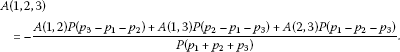(43)

On the other hand, if we consider the coefficient of ${\epsilon }^{4}$, knowing the fact that ${f}^{\left(4\right)}=0$, the perturbation equation (22) implies that

$2P\left(D\right)\left\{{f}^{\left(1\right)}\cdot {f}^{\left(3\right)}\right\}+P\left(D\right)\left\{{f}^{\left(2\right)}\cdot {f}^{\left(2\right)}\right\}=0.$

To be more precise, we have

$\begin{array}{c}{x}^{2{\alpha }_{1}+{\alpha }_{2}+{\alpha }_{3}}exp\left(\left(2{\beta }_{1}+{\beta }_{2}+{\beta }_{3}\right)t+\left(2{\eta }_{1}+{\eta }_{2}+{\eta }_{3}\right)\right)\hfill \\ \phantom{\rule{1em}{0ex}}×\left[A\left(1,2,3\right)P\left({p}_{2}+{p}_{3}\right)+A\left(1,2\right)A\left(1,3\right)P\left({p}_{2}-{p}_{3}\right)\right]\hfill \\ \phantom{\rule{1em}{0ex}}+{x}^{{\alpha }_{1}+2{\alpha }_{2}+{\alpha }_{3}}exp\left(\left({\beta }_{1}+2{\beta }_{2}+{\beta }_{3}\right)t+\left({\eta }_{1}+2{\eta }_{2}+{\eta }_{3}\right)\right)\hfill \\ \phantom{\rule{1em}{0ex}}×\left[A\left(1,2,3\right)P\left({p}_{1}+{p}_{3}\right)+A\left(1,2\right)A\left(2,3\right)P\left({p}_{1}-{p}_{3}\right)\right]\hfill \\ \phantom{\rule{1em}{0ex}}+{x}^{{\alpha }_{1}+{\alpha }_{2}+2{\alpha }_{3}}exp\left(\left({\beta }_{1}+{\beta }_{2}+2{\beta }_{3}\right)t+\left({\eta }_{1}+{\eta }_{2}+2{\eta }_{3}\right)\right)\hfill \\ \phantom{\rule{1em}{0ex}}×\left[A\left(1,2,3\right)P\left({p}_{1}+{p}_{2}\right)+A\left(1,3\right)A\left(2,3\right)P\left({p}_{1}-{p}_{2}\right)\right]=0.\hfill \end{array}$

The above expression is satisfied provided that

$A\left(1,2,3\right)=A\left(1,2\right)A\left(1,3\right)A\left(2,3\right).$
(44)

Since both expressions (43) and (44) for $A\left(1,2,3\right)$ should be equivalent, this equivalence gives rise to the fact that $P\left(D\right)$ given by (19) is not arbitrary but should satisfy Hirota condition (three-soliton solution condition) 

$\begin{array}{c}P\left({p}_{1}+{p}_{2}+{p}_{3}\right)P\left({p}_{1}-{p}_{2}\right)P\left({p}_{1}-{p}_{3}\right)P\left({p}_{2}-{p}_{3}\right)\hfill \\ \phantom{\rule{1em}{0ex}}+P\left({p}_{1}-{p}_{2}-{p}_{3}\right)P\left({p}_{1}+{p}_{2}\right)P\left({p}_{1}+{p}_{3}\right)P\left({p}_{2}-{p}_{3}\right)\hfill \\ \phantom{\rule{1em}{0ex}}+P\left({p}_{2}-{p}_{1}-{p}_{3}\right)P\left({p}_{1}+{p}_{2}\right)P\left({p}_{2}+{p}_{3}\right)P\left({p}_{1}-{p}_{3}\right)\hfill \\ \phantom{\rule{1em}{0ex}}+P\left({p}_{3}-{p}_{1}-{p}_{2}\right)P\left({p}_{1}+{p}_{3}\right)P\left({p}_{2}+{p}_{3}\right)P\left({p}_{1}-{p}_{2}\right)=0,\hfill \end{array}$

which can be written as

$\sum _{{\sigma }_{i}=±1}P\left(\sum _{i=1}^{3}{\sigma }_{i}{p}_{i}\right)\prod _{i
(45)

Here the multiplication is over all possible pairs of three elements such that $i. Therefore, since the parameters are restricted by the dispersion relations, the existence of a three-soliton solution is a constraint on P given by (45). Finally, the coefficients of ${\epsilon }^{j}$ for all $j⩾5$ vanish and the solution describing a head collision of three-q-solitons (see Figure 2) is expressed as

$\begin{array}{rcl}f\left(x,t\right)& =& 1+\sum _{i=1}^{3}{x}^{{\alpha }_{i}}exp\left({\beta }_{i}t+{\eta }_{i}\right)+\sum _{i
(46)

Remark 4.3 If $x\in {q}^{\mathbb{Z}}$, namely $x={q}^{n}$, $n\in \mathbb{Z}$, the differential-q-difference Toda equation (16) can be rewritten as

$\frac{{d}^{2}}{d{t}^{2}}log\left(1+V\left(x,t\right)\right)=V\left({q}^{n+1},t\right)+V\left({q}^{n-1},t\right)-2V\left(x,t\right),$
(47)

whose one-q-soliton solution turns out to be

$V\left(x,t\right)=\frac{{\beta }^{2}{q}^{n\alpha }exp\left(\beta t+\eta \right)}{{\left(1+{q}^{n\alpha }exp\left(\beta t+\eta \right)\right)}^{2}}.$
(48)

Subsequently, one can rewrite solutions describing two-q-solitons (35) and three-q-solitons (46).

## 5 The q-difference-q-difference Toda equation

In this section, we first answer the question of how to q-discretize the continuous time, in the light of q-difference operator (12). In order to propose the q-difference-q-difference Toda equation, we reverse the procedure and introduce a proper Hirota bilinear form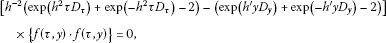(49)

which is a generalization of (19). Indeed, setting $\tau =exp\left(ht\right)$

${h}^{-2}\left(exp\left({h}^{2}\tau {D}_{\tau }\right)+exp\left(-{h}^{2}\tau {D}_{\tau }\right)-2\right)={h}^{-2}\left(exp\left(h{D}_{t}\right)+exp\left(-h{D}_{t}\right)-2\right),$
(50)

one can verify that the expression (50) tends to ${D}_{t}^{2}$ as $h\to 0$. Thus Hirota bilinear form (49) reduces to (19), from which we establish the q-difference-q-difference analogue of Toda equation. For convenience, we interchange ${h}^{2}$ by h in (49) and rewrite the Hirota bilinear form denoted by $P\left(D\right)$ as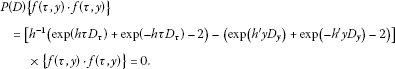(51)

By the virtue of the q-exponential identity (9), we may set

$exp\left(h\tau {D}_{\tau }\right)f\left(\tau ,y\right)f\left(\tau ,y\right)=f\left(q\tau ,y\right)f\left(\frac{\tau }{q},y\right),$
(52)
$exp\left({h}^{\prime }y{D}_{y}\right)f\left(\tau ,y\right)f\left(\tau ,y\right)=f\left(\tau ,{q}^{\prime }y\right)f\left(\tau ,\frac{y}{{q}^{\prime }}\right),$
(53)

equipped with ${e}^{h}=q$ and ${e}^{{h}^{\prime }}={q}^{\prime }$ respectively. Here we remark that nonuniform step sizes q and ${q}^{\prime }$ for the variables τ and y are different. Thus, Hirota bilinear form (51) turns out to be

${h}^{-1}\left[2f\left(q\tau ,y\right)f\left(\frac{\tau }{q},y\right)-2{f}^{2}\left(\tau ,y\right)\right]-\left(2f\left(\tau ,{q}^{\prime }y\right)f\left(\tau ,\frac{y}{{q}^{\prime }}\right)-2{f}^{2}\left(\tau ,y\right)\right)=0.$
(54)

We divide (54) by $2{f}^{2}\left(\tau ,y\right)$ and introduce the dependent variable transformation as

$V\left(\tau ,y\right):={h}^{-1}\left[\frac{f\left(q\tau ,y\right)f\left(\frac{\tau }{q},y\right)}{{f}^{2}\left(\tau ,y\right)}-1\right]=\frac{f\left(\tau ,{q}^{\prime }y\right)f\left(\tau ,\frac{y}{{q}^{\prime }}\right)}{{f}^{2}\left(\tau ,y\right)}-1.$
(55)

In order to construct the q-difference-q-difference analogue of Toda equation, we consider

$log\left(1+V\left(\tau ,y\right)\right)=logf\left(\tau ,{q}^{\prime }y\right)+logf\left(\tau ,\frac{y}{{q}^{\prime }}\right)-2logf\left(\tau ,y\right),$

from which one can encounter

${\mathrm{\Delta }}_{\tau }^{2}log\left(1+V\left(\tau ,y\right)\right)={\mathrm{\Delta }}_{y}^{2}\left({\mathrm{\Delta }}_{\tau }^{2}logf\left(\tau ,y\right)\right).$
(56)

On the other hand, if we consider

$log\left(1+hV\left(\tau ,y\right)\right)=logf\left(q\tau ,y\right)+logf\left(\frac{\tau }{q},y\right)-2logf\left(\tau ,y\right)={\mathrm{\Delta }}_{\tau }^{2}logf\left(\tau ,y\right).$
(57)

The equations (56) and (57) give rise to the desired q-difference-q-difference Toda equation

${\mathrm{\Delta }}_{\tau }^{2}log\left(1+V\left(\tau ,y\right)\right)={\mathrm{\Delta }}_{y}^{2}log\left(1+hV\left(\tau ,y\right)\right).$
(58)

For the rest of the discussion, to construct the three-q-soliton solutions of the equation (58), we utilize alike tools. Inserting the finite perturbation expansion (20) in Hirota bilinear form (51) and collecting the coefficients of ${\epsilon }^{i}$, $\mathrm{\forall }i⩾0$, the first coefficient is identically zero while the power of ${\epsilon }^{1}$ implies that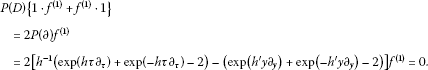(59)

Both space and time variables being q-discrete, by the Remark 4.1, it is possible to choose the starting solution of (59) as of the power form in both variables

${f}^{\left(1\right)}\left(\tau ,y\right)=\eta {\tau }^{\alpha }{y}^{\beta },$
(60)

where η, α are nonzero arbitrary constants and the related dispersion relation among the parameters is as follows:

${h}^{-1}\left({q}^{\alpha }+{q}^{-\alpha }-2\right)={\left({q}^{\prime }\right)}^{\beta }+{\left({q}^{\prime }\right)}^{-\beta }-2.$
(61)

Since all higher order perturbation parameters ${\epsilon }^{i}$, $i⩾2$ vanish by the assumption for i-soliton solution, ${f}^{\left(j\right)}=0$ for all $j⩾i+1$, we derive one-q-soliton solution using (55), (60) and (61) as

$V\left(\tau ,y\right)=\frac{\eta {\tau }^{\alpha }{y}^{\beta }\left[{\left({q}^{\prime }\right)}^{\beta }+{\left({q}^{\prime }\right)}^{-\beta }-2\right]}{{\left(1+\eta {\tau }^{\alpha }{y}^{\beta }\right)}^{2}}.$
(62)

If we choose the starting solution of (59) as

${f}^{\left(1\right)}\left(\tau ,y\right)={\eta }_{1}{\tau }^{{\alpha }_{1}}{y}^{{\beta }_{1}}+{\eta }_{2}{\tau }^{{\alpha }_{2}}{y}^{{\beta }_{2}},$
(63)

where ${\eta }_{i}$, ${\alpha }_{i}$ are arbitrary nonzero constants for all $i=1,2$, the coefficient of ${\epsilon }^{1}$ leads to the dispersion relation

${h}^{-1}\left({q}^{{\alpha }_{i}}+{q}^{-{\alpha }_{i}}-2\right)={\left({q}^{\prime }\right)}^{{\beta }_{i}}+{\left({q}^{\prime }\right)}^{-{\beta }_{i}}-2,\phantom{\rule{1em}{0ex}}\mathrm{\forall }i=1,2,$
(64)

and the solution describing collisions of two-q-solitons is determined as

$f\left(\tau ,y\right)=1+\sum _{i=1}^{2}{\eta }_{i}{\tau }^{{\alpha }_{i}}{y}^{{\beta }_{i}}+A\left(1,2\right){\eta }_{1}{\eta }_{2}{\tau }^{{\alpha }_{1}+{\alpha }_{2}}{y}^{{\beta }_{1}+{\beta }_{2}},$
(65)

what follows from the coefficient of ${\epsilon }^{2}$ allowing the form of ${f}^{\left(2\right)}$ as

${f}^{\left(2\right)}\left(\tau ,y\right)=A\left(1,2\right){\eta }_{1}{\eta }_{2}{\tau }^{{\alpha }_{1}+{\alpha }_{2}}{y}^{{\beta }_{1}+{\beta }_{2}},$
(66)

where the phase shift $A\left(1,2\right)$ among two-q-soliton solutions is expressed

$A\left(1,2\right)=-\frac{{h}^{-1}\left({q}^{{\alpha }_{1}-{\alpha }_{2}}+{q}^{{\alpha }_{2}-{\alpha }_{1}}-2\right)-\left({\left({q}^{\prime }\right)}^{{\beta }_{1}-{\beta }_{2}}+{\left({q}^{\prime }\right)}^{{\beta }_{2}-{\beta }_{1}}-2\right)}{{h}^{-1}\left({q}^{{\alpha }_{1}+{\alpha }_{2}}+{q}^{-{\alpha }_{1}-{\alpha }_{2}}-2\right)-\left({\left({q}^{\prime }\right)}^{{\beta }_{1}+{\beta }_{2}}+{\left({q}^{\prime }\right)}^{-{\beta }_{1}-{\beta }_{2}}-2\right)}=-\frac{P\left({p}_{1}-{p}_{2}\right)}{P\left({p}_{1}+{p}_{2}\right)},$
(67)

by means of vector notation ${p}_{1}±{p}_{2}=\left({\alpha }_{1}±{\alpha }_{2},{\beta }_{1}±{\beta }_{2},{\eta }_{1}±{\eta }_{2}\right)$. Here the coefficient of ${\epsilon }^{j}$ vanishes for all $j>3$, by the assumption ${f}^{\left(3\right)}=0$ and the dispersion relation (64) (see Figure 3).

For the three-q-soliton solution, choosing the starting solution of (59) as

${f}^{\left(1\right)}\left(\tau ,y\right)=\sum _{i=1}^{3}{\eta }_{i}{\tau }^{{\alpha }_{i}}{y}^{{\beta }_{i}},$
(68)

where ${\eta }_{i}$, ${\alpha }_{i}$ are arbitrary nonzero constants for all $i=1,2,3$, one can derive a similar dispersion relation from the coefficient of ${\epsilon }^{1}$

${h}^{-1}\left({q}^{{\alpha }_{i}}+{q}^{-{\alpha }_{i}}-2\right)={\left({q}^{\prime }\right)}^{{\beta }_{i}}+{\left({q}^{\prime }\right)}^{-{\beta }_{i}}-2,\phantom{\rule{1em}{0ex}}\mathrm{\forall }i=1,2,3,$
(69)

and the form of ${f}^{\left(2\right)}$

${f}^{\left(2\right)}\left(\tau ,y\right)=\sum _{i
(70)

which results from the coefficient of ${\epsilon }^{2}$. Here the interaction terms among the three-q-soliton solutions are

$A\left(i,j\right)=-\frac{{h}^{-1}\left({q}^{{\alpha }_{i}-{\alpha }_{j}}+{q}^{-\left({\alpha }_{i}-{\alpha }_{j}\right)}-2\right)-\left({\left({q}^{\prime }\right)}^{{\beta }_{i}-{\beta }_{j}}+{\left({q}^{\prime }\right)}^{-\left({\beta }_{i}-{\beta }_{j}\right)}-2\right)}{{h}^{-1}\left({q}^{{\alpha }_{i}+{\alpha }_{j}}+{q}^{-\left({\alpha }_{i}+{\alpha }_{j}\right)}-2\right)-\left({\left({q}^{\prime }\right)}^{{\beta }_{i}+{\beta }_{j}}+{\left({q}^{\prime }\right)}^{-\left({\beta }_{i}+{\beta }_{j}\right)}-2\right)}=-\frac{P\left({p}_{i}-{p}_{j}\right)}{P\left({p}_{i}+{p}_{j}\right)},$
(71)

for $i and $i,j=1,2,3$. The coefficient of ${\epsilon }^{3}$ leads to ${f}^{\left(3\right)}$ of the form

${f}^{\left(3\right)}\left(\tau ,y\right)=A\left(1,2,3\right){\eta }_{1}{\eta }_{2}{\eta }_{3}{\tau }^{{\alpha }_{1}+{\alpha }_{2}+{\alpha }_{3}}{y}^{{\beta }_{1}+{\beta }_{2}+{\beta }_{3}},$
(72)

where(73)

If the coefficient of ${\epsilon }^{4}$ is taken into account, since ${f}^{\left(4\right)}=0$, one can obtain another expression for $A\left(1,2,3\right)$

$A\left(1,2,3\right)=A\left(1,2\right)A\left(1,3\right)A\left(2,3\right),$
(74)

which should be equivalent to the form expressed in (73) provided that the inevitable Hirota condition (45) is satisfied. Finally, the solution describing the three-q-solitons (see Figure 4) is expressed in the form of a polynomial in power functions

$\begin{array}{rcl}f\left(x,t\right)& =& 1+\sum _{i=1}^{3}{\eta }_{i}{\tau }^{{\alpha }_{i}}{y}^{{\beta }_{i}}+\sum _{i
(75)

Remark 5.1 The q-difference-q-difference Toda equation (58) can be proposed in the form of

$\frac{\left(1+V\left(q\tau ,y\right)\right)\left(1+V\left(\frac{\tau }{q},y\right)\right)}{{\left(1+V\left(\tau ,y\right)\right)}^{2}}=\frac{\left(1+hV\left(\tau ,{q}^{\prime }y\right)\right)\left(1+hV\left(\tau ,\frac{y}{{q}^{\prime }}\right)\right)}{{\left(1+hV\left(\tau ,y\right)\right)}^{2}}.$
(76)

Here we remark that this form also exhibits the same dependent variable transformation (55). If

$\tau ={q}^{n},\phantom{\rule{2em}{0ex}}y={\left({q}^{\prime }\right)}^{m},\phantom{\rule{1em}{0ex}}n,m\in \mathbb{Z},$
(77)

it is possible to rewrite (76)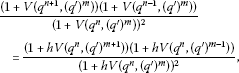(78)

whose solutions describing q-solitons (62), (65), (75) can be written by means of (77).

Remark 5.2 We present the graphs for differential-q-difference Toda equation and q-difference-q-difference Toda equation demonstrating two and three-q-soliton solutions respectively. We observe the solitonic behavior of the waves. Furthermore, being expressed as polynomials in power functions, we observe that the wave lengths of q-solitons increase as $|x|$ increases.

## 6 q-Hirota D-operator

In this section, we deal with a naturally arising question of q-differential version of Toda equation. In , the q-discrete version of two-dimensional Toda lattice equation, which is in a bilinear form reducing to two-dimensional Toda lattice equation as ${q}_{1},{q}_{2}\to 1$, is presented. The (τ functions) solutions are expressed in terms of Wronski determinant. Here, we present another q-discretized version of Toda equation in one dimension, the q-differential-q-difference Toda equation, and discuss the applicability of Hirota perturbation to produce its multi-soliton solutions. For that purpose, we first introduce the q-analogue of Hirota D-operator.

Definition 6.1 We define q-Hirota D-operator as

${D}_{q,x}^{m}\left\{f\cdot g\right\}:={\left({\partial }_{q,x}-{\partial }_{q,{x}^{\prime }}\right)}^{m}f\left(x\right)g\left({x}^{\prime }\right){|}_{{x}^{\prime }=x},$
(79)

where $f\left(x\right)$, $g\left(x\right)$ are arbitrary q-differentiable functions and $m\in {\mathbb{Z}}^{+}$.

Here ${\partial }_{q,x}$ is the q-derivative  acting as

${\partial }_{q,x}f\left(x\right)=\frac{f\left(qx\right)-f\left(x\right)}{\left(q-1\right)x}.$

We note that since ${\partial }_{q,x}\to {\partial }_{x}$ as $q\to 1$, q-Hirota D-operator ${D}_{q,x}\to {D}_{x}$ in the limit $q\to 1$. We present the properties of q-Hirota D-operator.

Proposition 6.2 Let$P\left({D}_{q}\right)$be a polynomial in${D}_{q,x}$, then

$\left(i\right)\phantom{\rule{1em}{0ex}}P\left({D}_{q}\right)\left\{f\cdot g\right\}=P\left(-{D}_{q}\right)\left\{g\cdot f\right\},$
(80)
$\left(ii\right)\phantom{\rule{1em}{0ex}}P\left({D}_{q}\right)\left\{f\cdot 1\right\}=P\left({\partial }_{q,x}\right)f;\phantom{\rule{2em}{0ex}}P\left({D}_{q}\right)\left\{1\cdot f\right\}=P\left(-{\partial }_{q,x}\right)f,$
(81)

where$f,g$are q-differentiable functions.

Proof For simplicity, consider the monomial $P\left({D}_{q}\right)={D}_{q,x}^{m}$. By definition (79),

$\begin{array}{rcl}P\left(-{D}_{q}\right)\left\{g\cdot f\right\}& =& {\left(-{D}_{q,x}\right)}^{m}g\left(x\right)f\left(x\right)={\left(-1\right)}^{m}\sum _{k=0}^{m}{\left(-1\right)}^{k}\left(\genfrac{}{}{0}{}{m}{k}\right){\partial }_{q,x}^{m-k}g\left(x\right){\partial }_{q,x}^{k}f\left(x\right)\\ =& {\left(-1\right)}^{m}f\left(x\right){\partial }_{q,x}^{m}g\left(x\right)+{\left(-1\right)}^{m-1}m{\partial }_{q,x}f\left(x\right){\partial }_{q,x}^{m-1}g\left(x\right)+\cdots +g\left(x\right){\partial }_{q,x}^{m}f\left(x\right)\\ =& P\left({D}_{q}\right)\left\{f\cdot g\right\},\end{array}$

where $\left(\genfrac{}{}{0}{}{m}{k}\right)$ is a classical binomial coefficient and ${\partial }_{q,x}^{i}$ is the i th q-derivative. As a consequence, setting $g\left(x\right)=1$, the property (81) results. □

We now propose the following equation:

$f\left(x,t\right){\partial }_{q,t}^{2}f\left(x,t\right)-{\left({\partial }_{q,t}f\left(x,t\right)\right)}^{2}-\left[f\left(qx,t\right)f\left(\frac{x}{q},t\right)-{f}^{2}\left(x,t\right)\right]=0,$
(82)

which admits the Hirota bilinear form

$P\left(D\right)\left\{f\left(x,t\right)\cdot f\left(x,t\right)\right\}=\left[{D}_{q,t}^{2}-\left(exp\left(hx{D}_{x}\right)+exp\left(-hx{D}_{x}\right)-2\right)\right]\left\{f\left(x,t\right)\cdot f\left(x,t\right)\right\}=0.$
(83)

Here we emphasize that Hirota bilinear form (83) reduces to the form (19) in the limit $q\to 1$. Thus, we may suppose (82) as a q-differential-q-difference version of Toda equation. If we utilize the perturbation expansion (20) in (21) equipped with $P\left(D\right)$, stated in (83), and Proposition 6.2, we encounter from the coefficient of ${\epsilon }^{1}$

$P\left(D\right)\left\{1\cdot {f}^{\left(1\right)}+{f}^{\left(1\right)}\cdot 1\right\}=2P\left(\partial \right){f}^{\left(1\right)}=2\left[{\partial }_{q,t}^{2}-\left(exp\left(hx{\partial }_{x}\right)+exp\left(-hx{\partial }_{x}\right)-2\right)\right]{f}^{\left(1\right)}=0.$
(84)

One can determine that

${f}^{\left(1\right)}\left(x,t\right)=\eta {x}^{\alpha }{e}_{q}^{\beta t},$
(85)

is a solution of the equation (84), provided that

${\beta }^{2}={q}^{\alpha }+{q}^{-\alpha }-2.$

Hence we face the same dispersion relation given in (25). Here η, α are nonzero arbitrary constants and ${e}_{q}^{t}$ is Jackson’s q-exponential function ,

${e}_{q}^{t}=\sum _{n=0}^{\mathrm{\infty }}\frac{{t}^{n}}{\left[n\right]!},$

where $\left[n\right]=1+q+{q}^{2}+\cdots +{q}^{n-1}$, $\left[n\right]!=\left[n\right]\cdot \left[n-1\right]\cdots \left[1\right]$, for all $n⩾1$ and $\left[0\right]!=1$. From the coefficient of ${\epsilon }^{2}$, we have

$P\left(D\right)\left\{1\cdot {f}^{\left(2\right)}+{f}^{\left(2\right)}\cdot 1+{f}^{\left(1\right)}\cdot {f}^{\left(1\right)}\right\}=2P\left(\partial \right){f}^{\left(2\right)}+P\left(D\right)\left\{{f}^{\left(1\right)}\cdot {f}^{\left(1\right)}\right\},$
(86)

which yields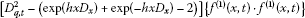(87)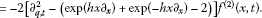(88)

Clearly ${f}^{\left(1\right)}$, given in (85), satisfies the equation (87) and thus we may assume ${f}^{\left(2\right)}=0$; as a consequence, we may assume for all higher order terms ${f}^{\left(j\right)}=0$, $j⩾2$. Indeed, we can choose a solution of (84) only of the form (85), which is discussed in the following remark.

Remark 6.3 If we choose the starting solution of (84) as

${f}^{\left(1\right)}\left(x,t\right)={\eta }_{1}{x}^{{\alpha }_{1}}{e}_{q}^{{\beta }_{1}t}+{\eta }_{2}{x}^{{\alpha }_{2}}{e}_{q}^{{\beta }_{2}t},$
(89)

from the coefficient of ${\epsilon }^{1}$, we obtain a relation for parameters

${\beta }_{i}^{2}={q}^{{\alpha }_{i}}+{q}^{-{\alpha }_{i}}-2,\phantom{\rule{1em}{0ex}}i=1,2,$

where ${\eta }_{i}$, ${\alpha }_{i}$ are nonzero arbitrary constants. From the coefficient of ${\epsilon }^{2}$, we have similarly (86) which implies

$P\left(\partial \right){f}^{\left(2\right)}=-{\eta }_{1}{\eta }_{2}\left[{\left({\beta }_{1}-{\beta }_{2}\right)}^{2}-\left({q}^{{\alpha }_{1}-{\alpha }_{2}}+{q}^{{\alpha }_{2}-{\alpha }_{1}}-2\right)\right]{x}^{{\alpha }_{1}+{\alpha }_{2}}{e}_{q}^{{\beta }_{1}t}{e}_{q}^{{\beta }_{2}t}.$
(90)

Note that there are two possible cases for ${f}^{\left(2\right)}$. If it is of the form ${f}^{\left(2\right)}=A\left(1,2\right){x}^{{\alpha }_{1}+{\alpha }_{2}}{e}_{q}^{{\beta }_{1}t+{\beta }_{2}t}$, then we need the additive property of q-exponentials

${e}_{q}^{z}{e}_{q}^{w}={e}_{q}^{z+w},$
(91)

which holds only if z, w are q-commuting variables, i.e., $wz=qzw$. In this case, this condition turns out to be ${\beta }_{1}{\beta }_{2}{t}^{2}=q{\beta }_{1}{\beta }_{2}{t}^{2}$, which implies immediately either ${\beta }_{1}=0$ (${\alpha }_{1}=0$) or ${\beta }_{2}=0$ (${\alpha }_{2}=0$). In other words, one of the summands declines in (89).

If ${f}^{\left(2\right)}$ is of the form $A\left(1,2\right){x}^{{\alpha }_{1}+{\alpha }_{2}}{e}_{q}^{{\beta }_{1}t}{e}_{q}^{{\beta }_{2}t}$, then because of the product rule ${\partial }_{q}\left(a\left(t\right)b\left(t\right)\right)=a\left(t\right){\partial }_{q}b\left(t\right)+b\left(qt\right){\partial }_{q}a\left(t\right)$ for q-derivative, the interaction term $A\left(1,2\right)$ is not independent of t.

To be more precise, although the equation (82) can be put into Hirota bilinear form (83), in both cases Hirota perturbation fails to produce further solutions. Moreover, it is straightforward to conclude that for any q-differential-q-difference or q-differential-difference type of equation even if the equation has a Hirota bilinear form, it is not possible to derive multisoliton solutions by the use of Hirota Direct method.

## References

1. Gardner CS, Greene JM, Kruskal MD, Miura RM: Method for solving the Korteweg-de Vries equation. Phys. Rev. Lett. 1967, 19: 1095–1097. 10.1103/PhysRevLett.19.1095

2. Wahlquist HD, Estabrook FB: Bäcklund transformation for solutions of the Korteweg-de Vries equation. Phys. Rev. Lett. 1973, 31: 1386–1390. 10.1103/PhysRevLett.31.1386

3. Hirota R: Exact solution of the Korteweg-de Vries equation for multiple collisions of solitons. Phys. Rev. Lett. 1971, 27: 1192–1194. 10.1103/PhysRevLett.27.1192

4. Hirota R: Exact solution of the modified Korteweg-de Vries equation for multiple collisions of solitons. J. Phys. Soc. Jpn. 1972, 33: 1456–1458. 10.1143/JPSJ.33.1456

5. Hirota R: Exact solution of the Sine-Gordon equation for multiple collisions of solitons. J. Phys. Soc. Jpn. 1972, 33: 1459–1463. 10.1143/JPSJ.33.1459

6. Hirota R: Exact envelope-soliton solutions of a nonlinear wave equation. J. Math. Phys. 1973, 14: 805–809. 10.1063/1.1666399

7. Grammaticos B, Ramani A, Hietarinta J: Multilinear operators: the natural extension of Hirota’s bilinear formalism. Phys. Lett. A 1994, 190: 65–70. 10.1016/0375-9601(94)90367-0

8. Hietarinta J: A search of bilinear equations passing Hirota’s three-soliton condition: I. KdV-type bilinear equations. J. Math. Phys. 1987, 28: 1732–1742. 10.1063/1.527815

9. Hietarinta J: Searching for integrable PDE’s by testing Hirota’s three-soliton condition. In Proceedings of the 1991 International Symposium on Symbolic and Algebraic Computation, ISSAC’91. Edited by: Watt SM. ACM, New York; 1991:295–300.

10. Gürses M, Pekcan A: 2+1 KdV(N) equations. J. Math. Phys. 2011., 52: Article ID 083516

11. Sato M: Soliton equations as dynamical systems on infinite dimensional Grassmann manifolds. RIMS Kokyuroku 1981, 439: 30–46.

12. Hirota R, Ohta Y, Satsuma J: Wronskian structures of solutions for soliton equations. Prog. Theor. Phys. Suppl. 1988, 94: 59–72.

13. Błaszak M, Gürses M, Silindir B, Szablikowski BM:Integrable discrete systems on $\mathbb{R}$ and related dispersionless systems. J. Math. Phys. 2008., 49: Article ID 072702

14. Hirota R: Exact N-soliton solution of a nonlinear lumped network equation. J. Phys. Soc. Jpn. 1973, 35: 286–288. 10.1143/JPSJ.35.286

15. Hirota R: Nonlinear partial difference equations. II. Discrete-time Toda equation. J. Phys. Soc. Jpn. 1977, 43: 2074–2078. 10.1143/JPSJ.43.2074

16. Hietarinta J: Introduction to the Hirota bilinear method. Springer Lecture Notes in Physics 495. In Integrability of Nonlinear Systems. Edited by: Kosman-Schwarzbach Y, Grammaticos B, Tamizhmani KM. Springer, Berlin; 1997:95–103.

17. Morikazu T: Vibration of a chain with nonlinear interaction. J. Phys. Soc. Jpn. 1967, 22: 431–436. 10.1143/JPSJ.22.431

18. Hirota R: The Direct Method in Soliton Theory. Cambridge University Press, Cambridge; 2004.

19. Kajiwara K, Satsuma J: q -difference version of two-dimensional Toda Lattice equation. J. Phys. Soc. Jpn. 1991, 60: 3986–3989. 10.1143/JPSJ.60.3986

20. Jackson FH: On q -functions and a certain difference operator. Trans. R. Soc. Edinb. 1908, 46: 253–281.

21. Jackson FH: A basic-sine and cosine with symbolical solution of certain differential equations. Proc. Edinb. Math. Soc. 1904, 22: 28–39.

22. Schützenberger MP:Une interprétation de certaines solutions de l’équation fonctionnelle: $F\left(x+y\right)=F\left(x\right)F\left(y\right)$. C. R. Acad. Sci. Paris 1953, 236: 352–353.

## Acknowledgement

The author would like to thank Prof. Maciej Błaszak for his valuable comments.

## Author information

Authors

### Corresponding author

Correspondence to Burcu Silindir.

### Competing interests

The author declares that she has no competing interests.

## Authors’ original submitted files for images

Below are the links to the authors’ original submitted files for images.

## Rights and permissions

Reprints and Permissions

Silindir, B. Soliton solutions of q-Toda lattice by Hirota direct method. Adv Differ Equ 2012, 121 (2012). https://doi.org/10.1186/1687-1847-2012-121

• Accepted:

• Published:

• DOI: https://doi.org/10.1186/1687-1847-2012-121

### Keywords

• Hirota direct method
• q-Toda lattice
• q-soliton solutions
• q-exponential identity
• q-Hirota D-operator